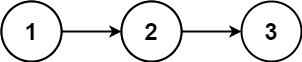# Leetcode 382 - Linked List Random Node

Note:

• Assume there is lots of data and you don’t know about the length of it. How to pick one randomly in O(N)?
• Use reservoir sampling!
• Conclusion: The possibility of num with index i to be picked is k / N . (n is the count of all nums so far. k is how many nums we want to pick)
• Proof:
•• Detail:
1. When i > k, pick a random number between [1, i].
2. If ran < K, it means we randomly picked an interval with length k. The possibility of it is k / n. So we need to keep the cur.
3. But which one should be replaced?
4. The possibility to picked one from result[] is also 1 / k.
5. Now that ran is between [1, K]. Let’s replace k[ran] with k[i] coz we picked k[ran] among k elements with the possibility of 1 / k.

Question:

Given a singly linked list, return a random node’s value from the linked list. Each node must have the same probability of being chosen.

Implement the Solution class:

• Solution(ListNode head) Initializes the object with the integer array nums.
• int getRandom() Chooses a node randomly from the list and returns its value. All the nodes of the list should be equally likely to be choosen.

Example:Code: